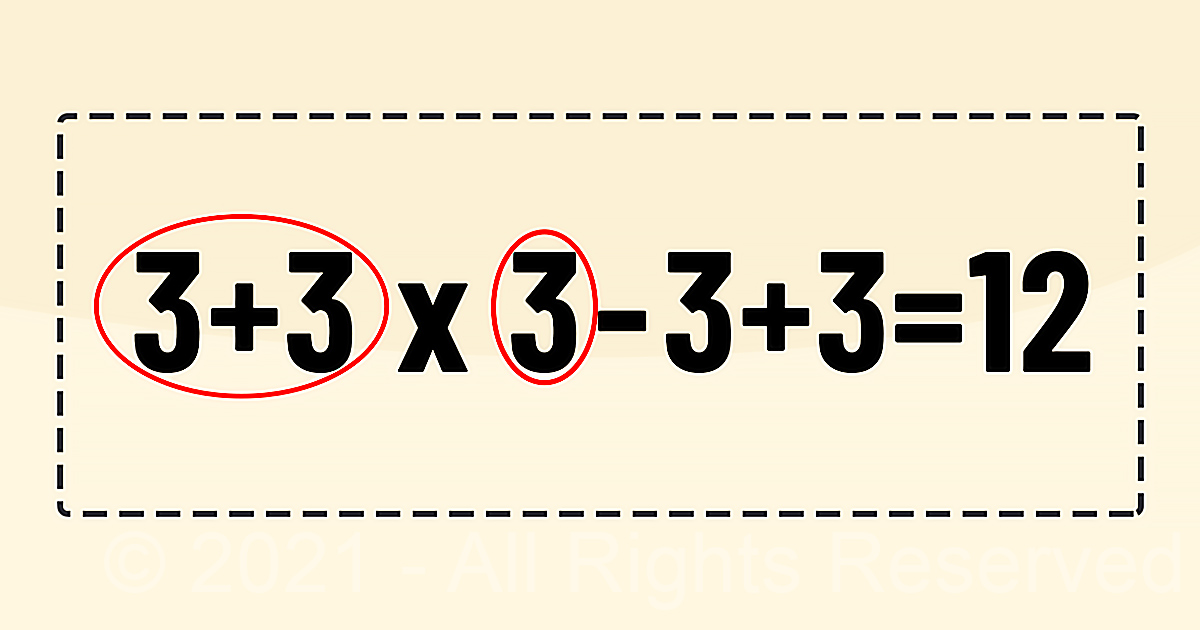# Most people are stumped by this simple math problemMath is not as hard as it looks. You only need to know the right order of operations to become an expert. These math tips will help you to improve your math skills.

This math equation may look simple, but if you don’t follow the right order of math operations, you will never solve it. So, you have to use PEMDAS, in other words, parentheses, exponents, multiplication, division, addition, subtraction and in that order.

Here we have the equation 3+3 x 3-3+3=? It looks simple at first, but if you don’t follow the right order of the equation, you might not get it.Let’s see how it works: Again, the right order of operation to use is PEMDAS which stands for parentheses, exponents, multiplication, division, addition, subtraction.

Since there are no parentheses or exponents, you would use multiplication. 3 x 3 equals 9. Next, you follow it with addition, 9 + 3 = 12.

Then, you subtract 3 from 12, and that takes you back to 9. Lastly, you simply add the last 3 to 9, and you get 12.